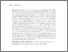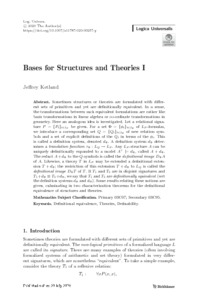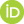# Bases for Structures and Theories I

Ketland, Jeffrey (2020) Bases for Structures and Theories I. Logica Universalis, 14. pp. 357-381.Preview
Text
Ketland2020_Article_BasesForStructuresAndTheoriesI.pdf

## Abstract

Sometimes structures or theories are formulated with different sets of primitives and yet are definitionally equivalent. In a sense, the transformations between such equivalent formulations are rather like basis transformations in linear algebra or co-ordinate transformations in geometry. Here an analogous idea is investigated. Let a relational signature $P = \{P_i\}_{i \in I_P}$ be given. For a set $\Phi = \{\phi_i\}_{i \in I_{\Phi}}$ of $L_P$-formulas, we introduce a corresponding set $Q = \{Q_i\}_{i \in I_{\Phi}}$ of new relation symbols and a set of explicit definitions of the $Q_i$ in terms of the $\phi_i$. This is called a definition system, denoted $d_{\Phi}$. A definition system $d_{\Phi}$ determines a \emph{translation function} $\tau_{\Phi} : L_Q \to L_P$. Any $L_P$-structure $A$ can be uniquely definitionally expanded to a model $A^{+} \models d_{\Phi}$, called $A + d_{\Phi}$. The reduct $A + d_{\Phi}$ to the $Q$-symbols is called the \emph{definitional image} $D_{\Phi}A$ of $A$. Likewise, a theory $T$ in $L_P$ may be extended a definitional extension $T + d_{\Phi}$; the restriction of this extension $T + d_{\Phi}$ to $L_Q$ is called the \emph{definitional image} $D_{\Phi}T$ of $T$. If $T_1$ and $T_2$ are in disjoint signatures and $T_1 + d_{\Phi} \equiv T_2 + d_{\Theta}$, we say that $T_1$ and $T_2$ are \emph{definitionally equivalent} (wrt the definition systems $d_{\Phi}$ and $d_{\Theta}$). Some results relating these notions are given, culminating in two characterization theorems for the definitional equivalence of structures and theories.

 Export/Citation: EndNote | BibTeX | Dublin Core | ASCII/Text Citation (Chicago) | HTML Citation | OpenURL
 Social Networking:

Item Type: Published Article or Volume
Creators:
CreatorsEmailORCIDKetland, Jeffreyjeffreyketland@gmail.com0000-0002-5128-4387
Keywords: Definitional equivalence; theoretical equivalence
Subjects: General Issues > Structure of Theories
Depositing User: Dr Jeffrey Ketland
Date Deposited: 29 Nov 2020 04:26
Item ID: 18458
Journal or Publication Title: Logica Universalis
Publisher: Springer
DOI or Unique Handle: https://doi.org/10.1007/s11787-020-00257-y
Subjects: General Issues > Structure of Theories
Date: 2020
Page Range: pp. 357-381
Volume: 14
URI: http://philsci-archive.pitt.edu/id/eprint/18458

### Monthly Views for the past 3 yearsView Item